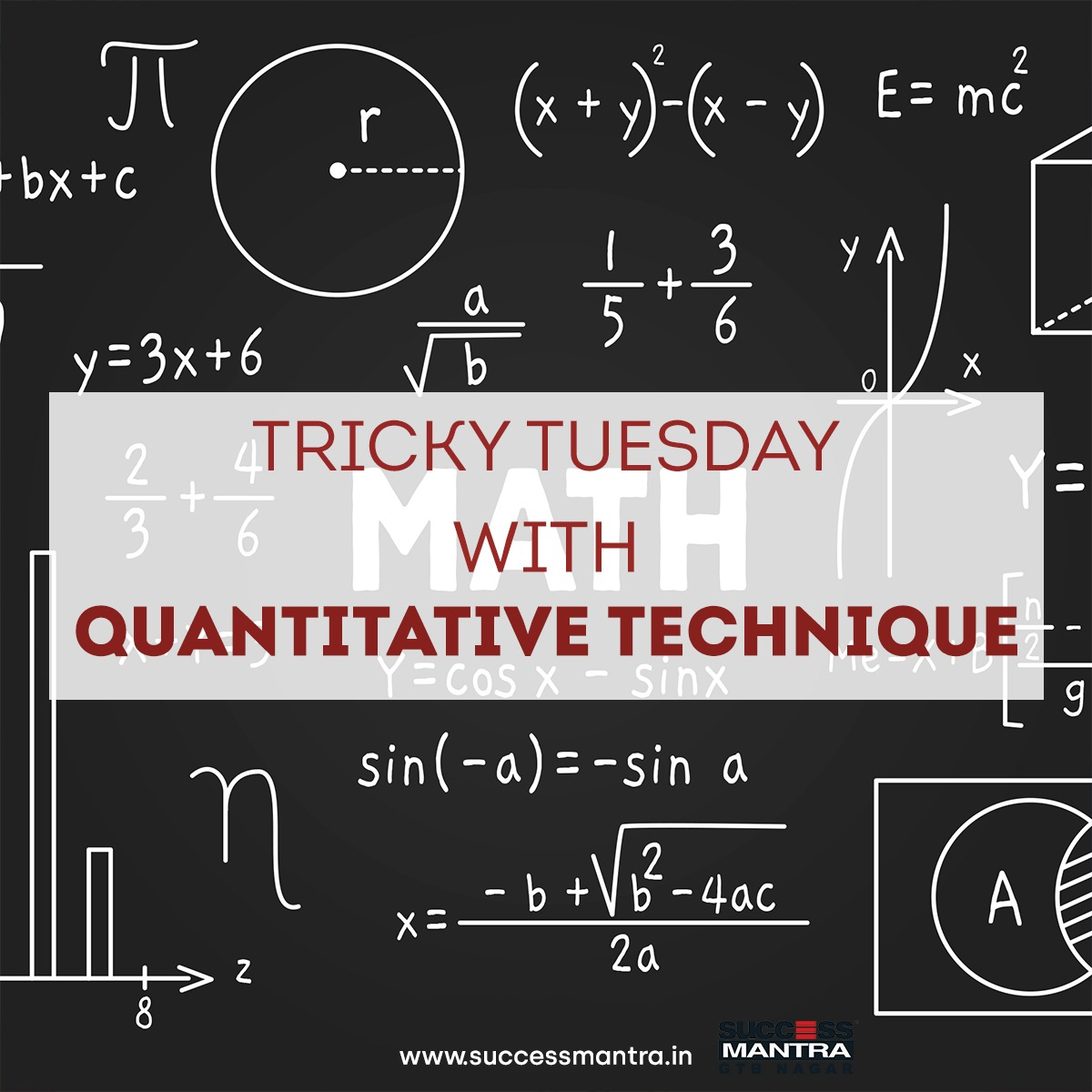## Questions On Quantitative Techniques SMQTQ0291. 720 sweets were distributed equally among children in such a way that the number of sweets received by each child is 20% of the total number of children. How many sweets did each child receive?

(a) 8
(b) 10
(c) 9
(d) 12

2. How many liters of a 30% alcohol solution should be added to 40 liters of a 60% alcohol solution to prepare a 50% solution?

(a) 30
(b) 20
(c) 24
(d) 32

3. What least value must be assigned to ‘*’ so that the number 63576 * 2 is divisible by 8?

(a) 1
(b) 2
(c) 3
(d) 4

4. When a number is divided by 5, the remainder is 3. What will be the remainder when sum of cube of that number and square of that number is divided by 5?

(a) 1
(b) 2
(c) 3
(d) 4

5. Arun sold 18 toys for Rs 16800 gaining there by the cost price of 3 toys. Find the cost price of a toy?

(a) 900
(b) 1200
(c) 800
(d) 600

1. D
2. B
3. C
4. A
5. C